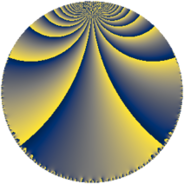# Properties

 Label 304.5.lLevel $304$ Weight $5$ Character orbit 304.l Rep. character $\chi_{304}(115,\cdot)$ Character field $\Q(\zeta_{4})$ Dimension $288$ Sturm bound $200$

# Related objects

## Defining parameters

 Level: $$N$$ $$=$$ $$304 = 2^{4} \cdot 19$$ Weight: $$k$$ $$=$$ $$5$$ Character orbit: $$[\chi]$$ $$=$$ 304.l (of order $$4$$ and degree $$2$$) Character conductor: $$\operatorname{cond}(\chi)$$ $$=$$ $$16$$ Character field: $$\Q(i)$$ Sturm bound: $$200$$

## Dimensions

The following table gives the dimensions of various subspaces of $$M_{5}(304, [\chi])$$.

Total New Old
Modular forms 324 288 36
Cusp forms 316 288 28
Eisenstein series 8 0 8

## Trace form

 $$288q + 12q^{4} - 132q^{6} + O(q^{10})$$ $$288q + 12q^{4} - 132q^{6} - 192q^{11} + 660q^{12} - 156q^{14} - 620q^{16} - 2720q^{18} - 1200q^{20} - 1804q^{22} - 2304q^{23} + 612q^{24} + 2064q^{26} + 3648q^{27} + 3720q^{28} - 1728q^{29} + 5384q^{30} - 3060q^{32} - 6880q^{34} - 15868q^{36} + 3648q^{37} - 5376q^{39} + 10104q^{40} + 17140q^{42} - 3392q^{43} + 10020q^{44} - 620q^{48} + 98784q^{49} - 24696q^{50} + 13760q^{51} - 28268q^{52} + 960q^{53} - 5992q^{54} + 23552q^{55} + 12348q^{56} + 31920q^{58} + 5568q^{59} + 27040q^{60} + 38556q^{62} - 8064q^{64} + 4032q^{65} - 39796q^{66} - 18880q^{67} - 26472q^{68} - 1284q^{70} - 39936q^{71} + 43788q^{72} + 18744q^{74} - 2304q^{75} + 9408q^{77} + 48436q^{78} - 7128q^{80} - 209952q^{81} + 14428q^{82} + 10560q^{83} - 48284q^{84} + 37140q^{86} - 64840q^{88} - 13544q^{90} + 46464q^{91} + 36552q^{92} - 17472q^{93} + 5496q^{94} + 6584q^{96} + 5520q^{98} - 49216q^{99} + O(q^{100})$$

## Decomposition of $$S_{5}^{\mathrm{new}}(304, [\chi])$$ into newform subspaces

The newforms in this space have not yet been added to the LMFDB.

## Decomposition of $$S_{5}^{\mathrm{old}}(304, [\chi])$$ into lower level spaces

$$S_{5}^{\mathrm{old}}(304, [\chi]) \cong$$ $$S_{5}^{\mathrm{new}}(16, [\chi])$$$$^{\oplus 2}$$